iamluisro.coCoding Challenge: Palindromes

I recently started doing coding challenges to prepare for job interviews. And I've still got many more coding challenges to go and much more to learn about computer science. This is one vast field of study, with too much to cover in such little time. But we do what we can with the time we have! So I decided to write some brief posts on my experience solving some of these problems.

Finding whether a string is a palindrome or not is a simple problem that can get really complex really quickly. Here's a quick and simple way of tackling this problem.

## Problem

Problem: Find whether a string is a palindrome or not. Language: JavaScript; Input: 'hello', 'civic', 'tattarrattat';
Output: false, true, true (respectively) ;

## Solution:

### Using existing methods

We can start out our function using an arrow function, though you can use your choice of a declarative function or your own expression function. Arrow Functions are the new thing, so let's use it and be familiar with them.

``````const palindrome = (str) => {

}
palindrome('civic');
``````

That's a basic setup and we'll call the function with the word 'civic' to start out with.

If we manually check to see if the word 'civic' is a palindrome we find the answer to be true.

Forward: 'c', 'i', 'v', 'i', 'c' ; Reverse: 'c', 'i', 'v', 'i', 'c' ;

Awesome. So, our answer should be true! Let's move on to next the step.

What we first want to do is be able to manage the string. Here's where we can use our good friend, the .split() method, helping us turn the string into an array.

``````const palindrome = (str) => {
const arr = str.split('');
}
palindrome('civic');
``````

Here we assign the constant variable "arr" the value of our string that has been split by each space of a character.

If we take our word 'civic' and split it, the value of our const 'arr' will now look like this:

``````arr = ['c', 'i', 'v', 'i', 'c'];
``````

With our string now transformed into an array, we can now modify it and transform it further.

From here, things can get more complex, but let's cover a very simple solution.

We'll use the .reverse() method which is already meant to help us do what its name suggests: reverse an array. And lucky for us, we already have an array from our string, otherwise we wouldn't be able to do anything with that string.

Applying the .reverse() method looks like this:

``````const palindrome = (str) => {
const arr = str.split('');
arr.reverse()
}
palindrome('civic');
``````

Now that we have reversed our string, it's going to look a little something like this:

``````arrReversed = ['c', 'i', 'v', 'i', 'c'];
``````

Of course it'll look the same. If our initial string were ('hello'), the array would look like this:

``````arr = ['o', 'l', 'l', 'e', 'h'];
``````

Now what we need to do is join this array. If you remember, we split the array as one of our first steps, so we need to join the reversed array in order to finally compare the initial string to the reversed string.

We can join the reversed array with the .join() method.

``````const palindrome = (str) => {
const arr = str.split('');
let reversedString = arr.reverse().join('');
}
palindrome('civic');
``````

The .join() method accepts a parameter that will be our separator. In this case, we use `('')` to tell the .join() method we're going to join the elements in the array without any characters or spaces between them. Now we'll assign the reversed string into the variable using a "let" JavaScript keyword named "reversedString" .

Finally, we need to compare whether our reversedString is the same as our initial string. What we need to do is use a condition expression to see if reversedString is equal to our initial string.

``````const palindrome = (str) => {
const arr = str.split('');
let reversedString = arr.reverse().join('');
if (reversedString === str) {
console.log(true);
} else {
console.log(false);
}
}
palindrome('civic');
``````

The above expression can also be written using a Ternary Operator.

``````const palindrome = (str) => {
const arr = str.split('');
let reversedString = arr.reverse().join('');
(reversedString === str) ? console.log(true) : console.log(false);
}
palindrome('civic');
``````

For the string 'civic' we get our expected result of "true" and a runtime of .088 seconds.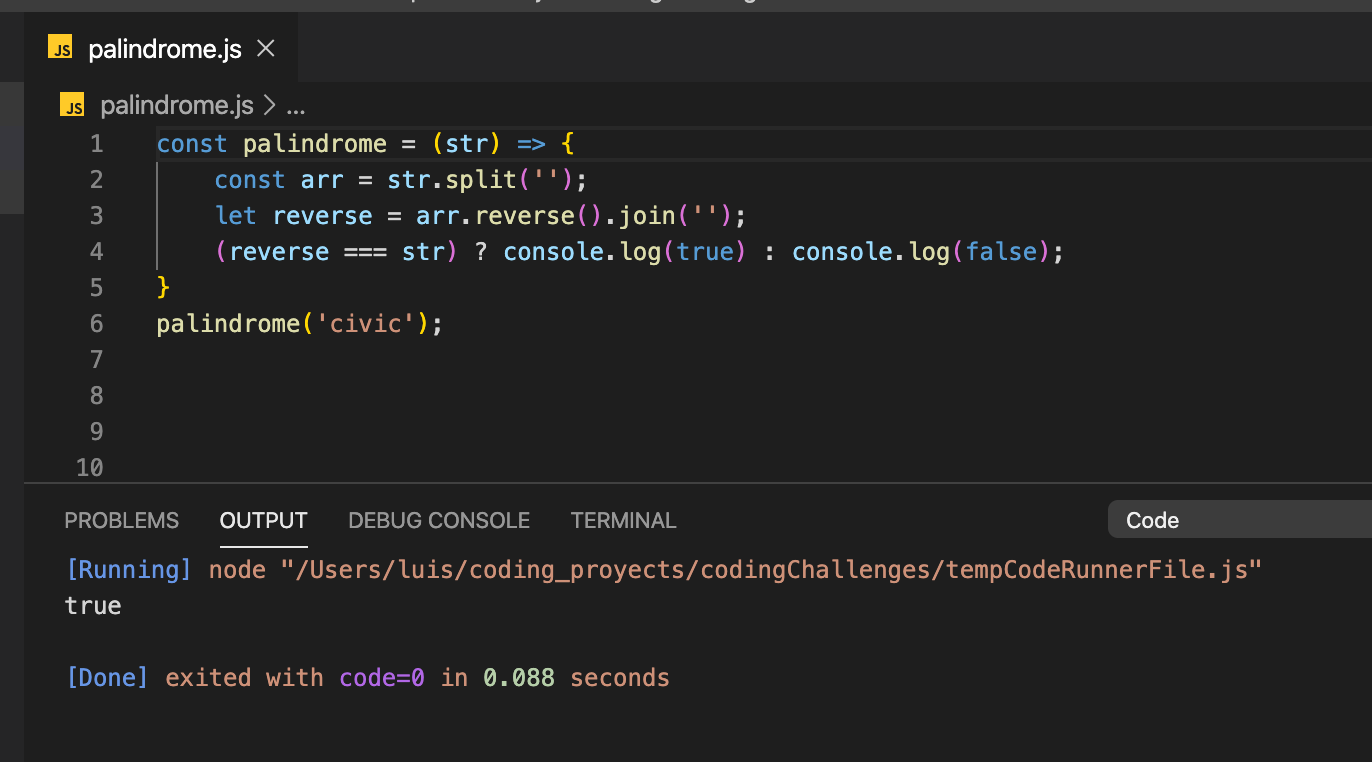For the string 'hello' we get our expected result of "false" and a runtime of .275 seconds.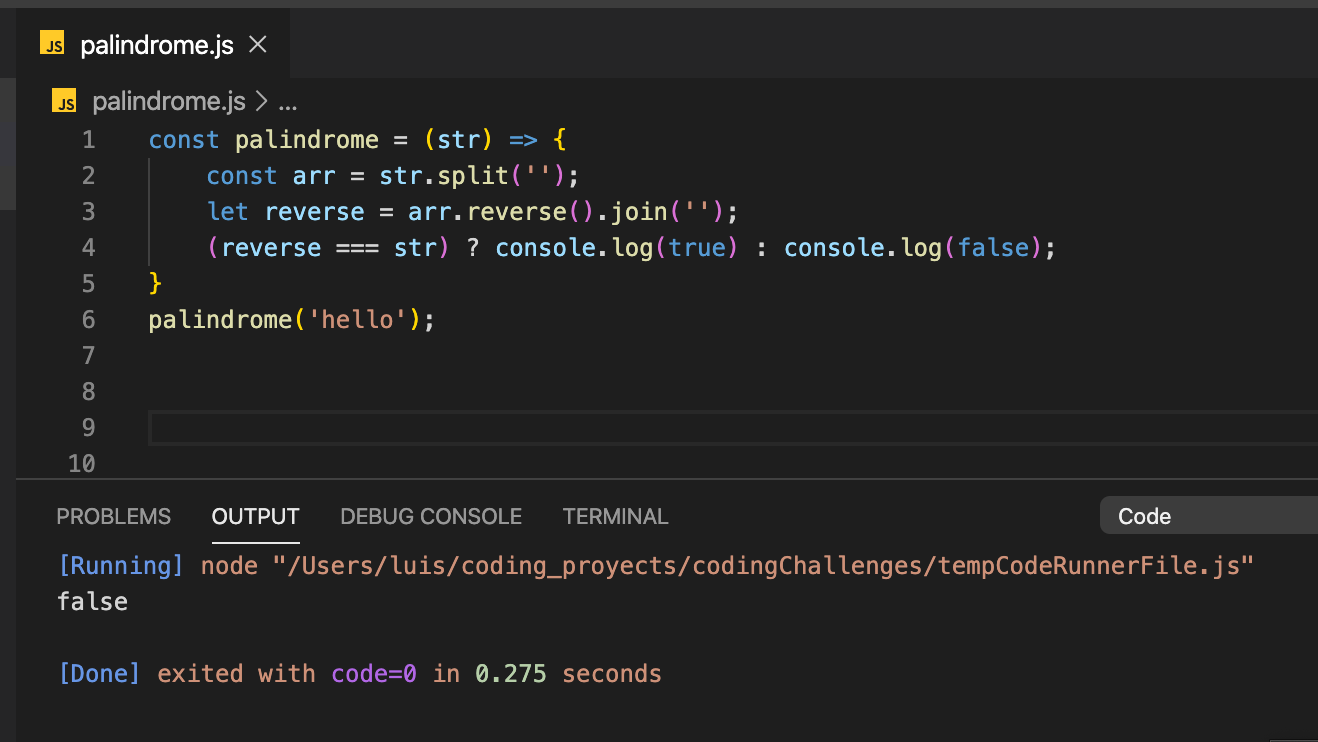Finally, for the string 'tattarrattat' we get our expected result of "false" and a runtime of .217 seconds.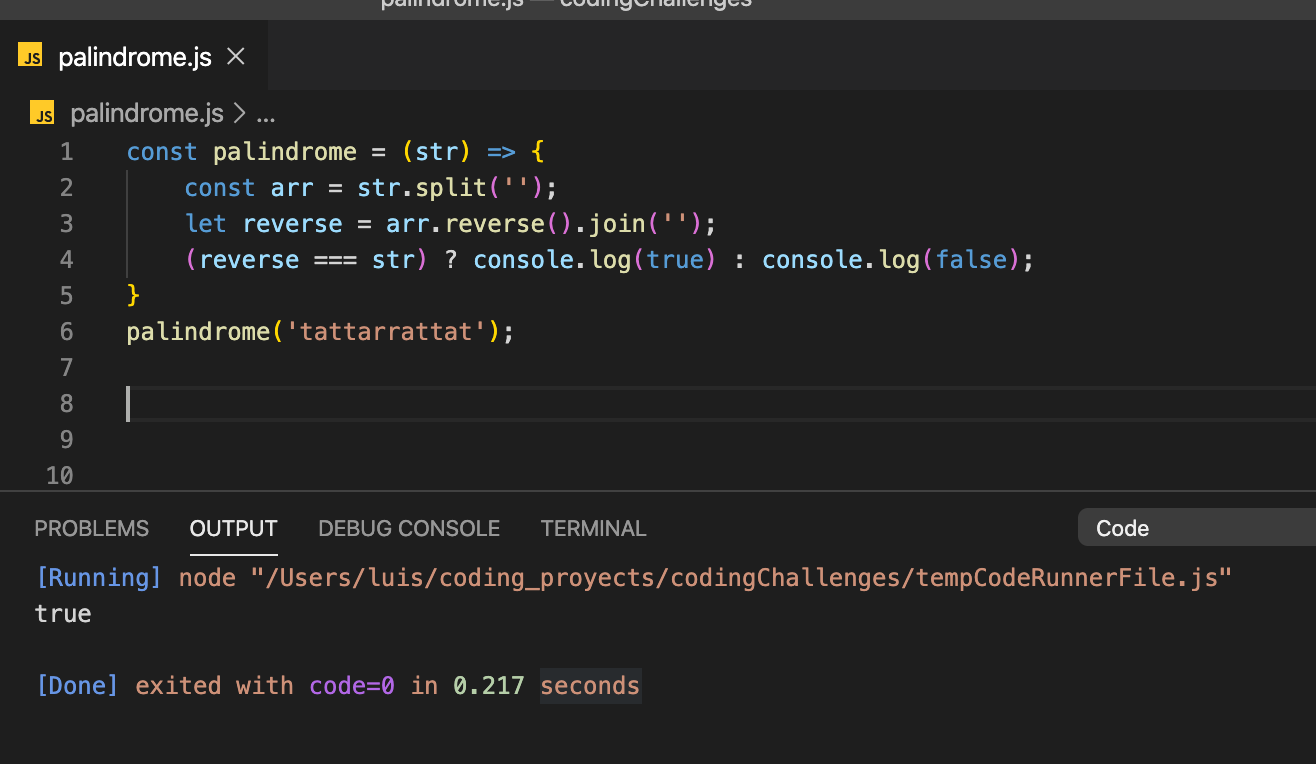Great! This was the simple way of solving this problem.

### Using a FOR Loop

One other way I'd like to cover in this post is using a FOR loop. Our goal will be to use the For loop to insert the string in reverse to the "reverse" variable. And finally, we will compare the "reverse" variable with the original string.

We start out declaring a and initialize it as an empty string. In my case, I assign the "reverse" variable the empty string.

``````const palindrome = (str) => {
let reverse = '';

}
palindrome('civic');
``````

We proceed to initializing our For loop, where "i" will be the position / index of a character of the string.

``````const palindrome = (str) => {
let reverse = '';
for (i = str.length - 1; i = 0; i--) {
};

}
palindrome('civic');
``````

A couple of things happened here. We start out with i being equal to the string's length minus 1. We subtract 1 from the string's length because we want to avoid having an undefined value. If we have `i = str.length`, we'd be iterating into a space where there is no character to read, giving us an undefined value. If we do length of the previous string's we've used ('hello', 'civic' or 'tattarrattat'), we'd get the following responses:

``````'civic'.length = 5;
'hello'.length = 5;
'tattarrattat'.length = 12;
``````

However, if we look for a character in 'civic' by using the following syntax, `str` we'd get `undefined`. To get the last character, 'c', we'd need to have `str`, hence the `str.length - 1`.

Next, since we're having the string be input into the "reverse" variable in rever, we're starting with the last character of the string and iterating until we reach the first character of the string. In other words, until we reach str. This is why we have ì >= 0.

Like I mentioned before, we're starting at the last character and moving to the first character. In order to do this, we need to decrease our "i" variable by 1 until we reach "0". We tell the for loop to decrease by 1 by using "i--" .

Awesome. Now that we have our for loop established, we need to tell the program what to do. And what we'll we do is insert the characters as they come into the "reverse" variable we previously initialized as an empty string.

In order to do that, we use the "+=" operator.

``````const palindrome = (str) => {
let reverse = '';
for (i = str.length - 1; i = 0; i--) {
reverse += str[i];
};

}
palindrome('civic');
``````

Just to clarify, we're inserting the characters of the string in reverse order. That looks like:

``````For 'hello'
reverse += str = 'o';
reverse += str = 'l';
reverse += str = 'l';
reverse += str = 'e';
reverse += str = 'h';
``````

And we have reverse = 'olleh'. The reverse!

Now that we have the reverse, let's compare it to the original string! And we can do that simply like in our example above.

```````(reverse === str) ? console.log(true) : console.log(false)`
``````

Our code will now look like this:

``````const palindrome = str => {
var reverse = '';
for (i = str.length - 1; i >= 0; i--) {
reverse += str[i]
};

(reverse === str) ? console.log(true) : console.log(false);
}
``````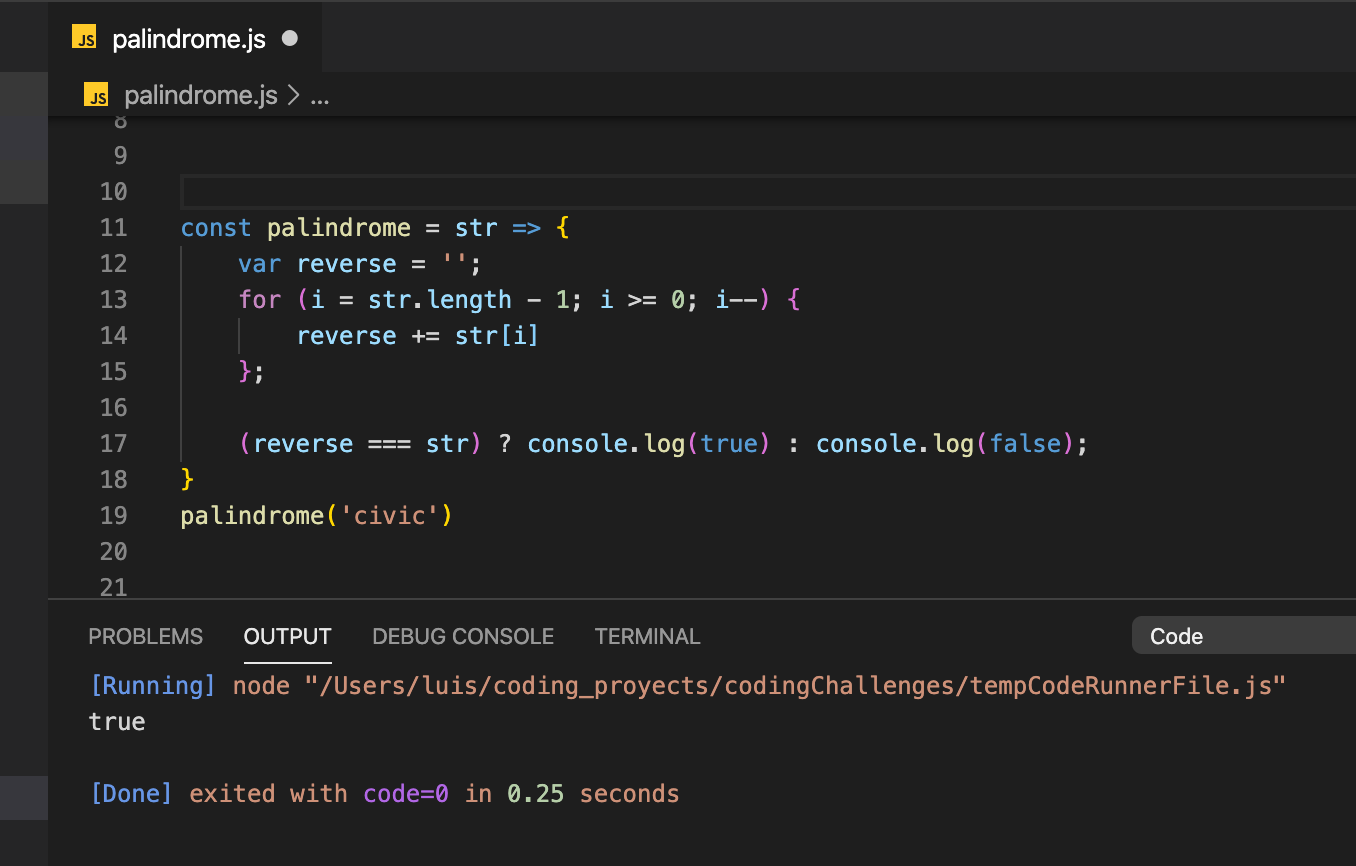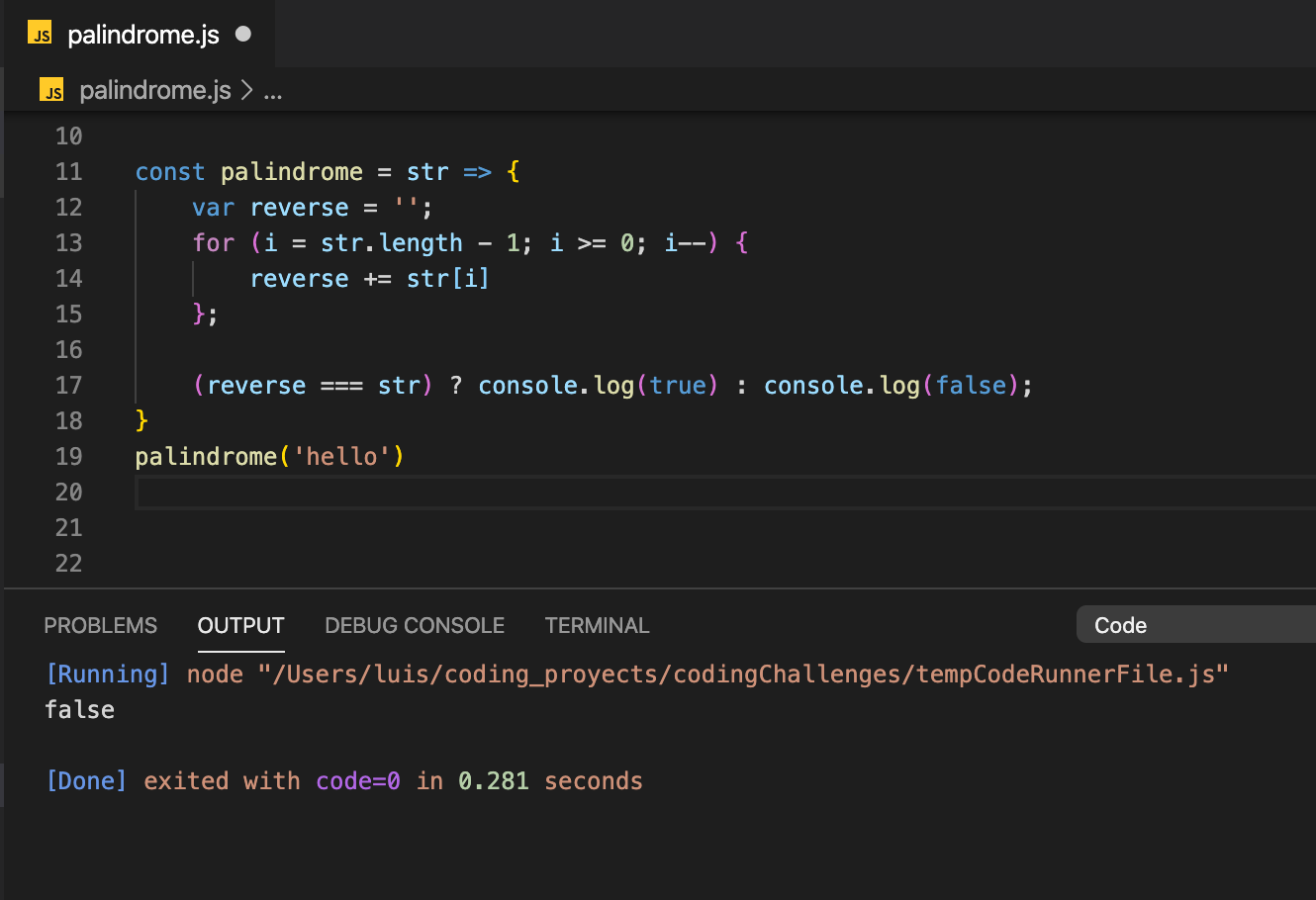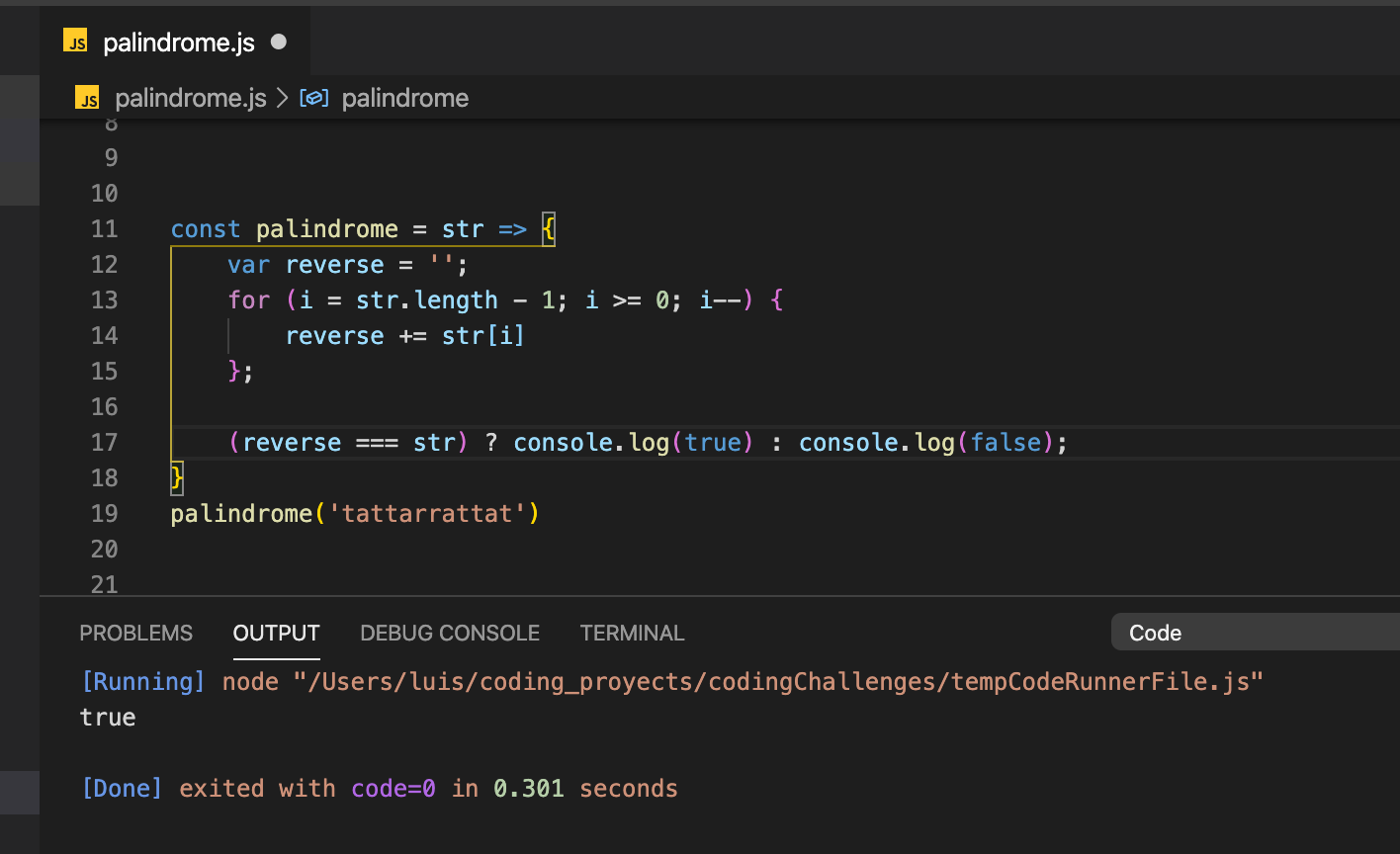## Conclusion

There are plenty more ways to solve this particular problem. Solve are more efficient and quicker, others can be a bit more sluggish. There are also different variations of the question. It's all a matter of perspective and what the problem is asking.

What I shared here are two simple ways to start finding if a string is a palindrome or not.

I'll keep diving deeper into algorithms and efficiency and will circle back to share better solutions.

Until next time! And thanks for reading.

Tags:
javascript